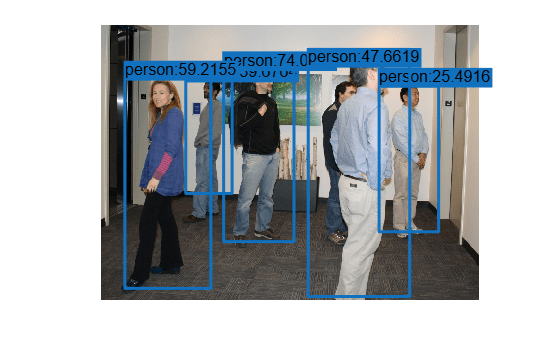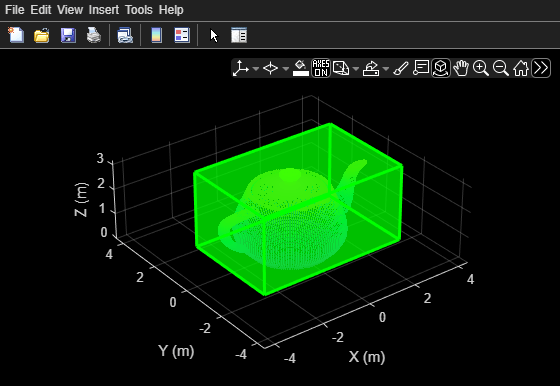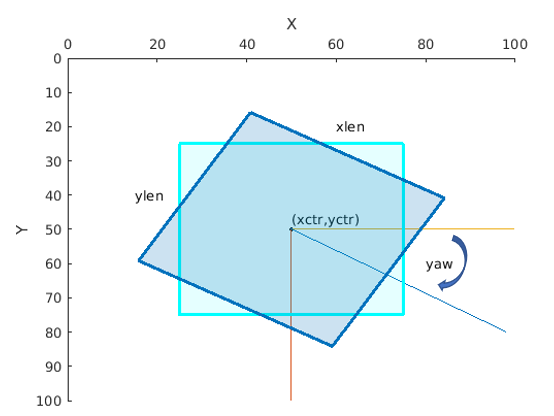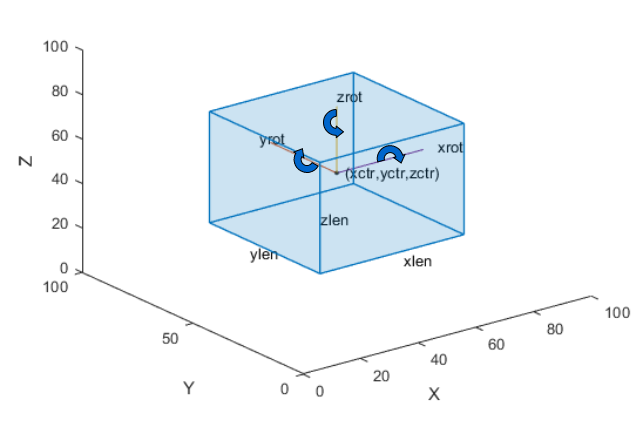showShape

Display shapes on image, video, or point cloud

Description

example

showShape(shape,position) displays one or more instances of a shape shape in the current axes at the specified locations position.

example

showShape(___,Name,Value) specifies options using one or more name-value pair arguments. For example, 'Color','yellow' specifies the color of the displayed shapes as yellow.

Examples

collapse all

Read an image into the workspace.

Create an aggregate channel features (ACF) people detector.

detector = peopleDetectorACF()
detector =
acfObjectDetector with properties:

ModelName: 'inria-100x41'
ObjectTrainingSize: [100 41]
NumWeakLearners: 2048

Detect people in the image.

[bboxes,scores] = detect(detector,I);

Display the image with a labeled rectangle, including the associated detection score, around each detected person.

figure
imshow(I)
labels = "person:"+scores;
showShape('rectangle',bboxes,'Label',labels)Read point cloud data into the workspace.

Display the point cloud data.

figure
pcshow(ptCloud)
xlabel('X')
ylabel('Y')
zlabel('Z')

Define a cuboid and display it in green with an opacity of 0.5.

pos = [0.3753 0 1.65 6 4 3 0 0 0];
showShape('cuboid',pos,'Color','green','Opacity',0.5)Read point cloud data into the workspace.

Define a rotation matrix and 3-D transform to rotate the point cloud, and an associated cuboid, by 5 degrees.

rot = 5;
R = [ cosd(rot) sind(rot) 0 0; ...
-sind(rot) cosd(rot) 0 0; ...
0       0           1 0; ...
0       0           0 1];

tform = affine3d(R);

Compute the x and y rendered limits of the point cloud to ensure that the point cloud is not clipped during rotation.

pcLimits = abs([ptCloud.XLimits ptCloud.YLimits]);
maxLimit = max(pcLimits);

Add an additional margin to the plot to prevent the cuboid from being clipped during rotation.

margin = 1;
maxLimit = maxLimit + margin;

xlimits = [-maxLimit maxLimit];
ylimits = [-maxLimit maxLimit];
zlimits = ptCloud.ZLimits;

Create a player for visualizing the point cloud.

player = pcplayer(xlimits,ylimits,zlimits);

Customize the player axis labels.

xlabel(player.Axes,'X (m)');
ylabel(player.Axes,'Y (m)');
zlabel(player.Axes,'Z (m)');

Define a cuboid around the point cloud.

cuboidPosition = [0.3753 0 1.65 6 4 3 0 0 0];

Define the output view for a cuboid rotation. Use the same limits as the player so that the cuboid is not clipped. Then, display the rotated point cloud and cuboid.

gridSize = [1 1 1];
ref = imref3d(gridSize,xlimits,ylimits,zlimits);

for i = 1:round((360/rot))
% Rotate point cloud.
ptCloud = pctransform(ptCloud,tform);

% Rotate the cuboid.
cuboidPosition = bboxwarp(cuboidPosition,tform,ref);

% Show rotated point cloud data.
view(player,ptCloud)

% Show rotated cuboid.
showShape('cuboid',cuboidPosition, ...
'Parent',player.Axes, ...
'Color','green', ...
'Opacity',0.5)

% Use drawnow to synchronize point cloud and shape visualization.
drawnow
endInput Arguments

collapse all

Type of shape, specified as a character vector. The vector can be 'line', 'rectangle', 'cuboid, 'polygon', or 'circle'.

Data Types: string

Positions and sizes of shapes, specified according to the type of shapes, described in this table.

Bounding BoxDescription
Axis-aligned rectangle

Defined in pixel coordinates as an M-by-4 numeric matrix with rows of the form [x y w h], where:

• M is the number of axis-aligned rectangles.

• x and y specify the upper-left corner of the rectangle.

• w specifies the width of the rectangle, which is its length along the x-axis.

• h specifies the height of the rectangle, which is its length along the y-axis.

Rotated rectangle

Defined in spatial coordinates as an M-by-5 numeric matrix with rows of the form [xctr yctr xlen ylen yaw], where:

• M is the number of rotated rectangles.

• xctr and yctr specify the center of the rectangle.

• xlen specifies the width of the rectangle, which is its length along the x-axis before rotation.

• ylen specifies the height of the rectangle, which is its length along the y-axis before rotation.

• yaw specifies the rotation angle in degrees. The rotation is clockwise-positive around the center of the bounding box.Cuboid

Defined in spatial coordinates as an M-by-9 numeric matrix with rows of the form [xctr yctr zctr xlen ylen zlen xrot yrot zrot], where:

• M is the number of cuboids.

• xctr, yctr, and zctr specify the center of the cuboid.

• xlen, ylen, and zlen specify the length of the cuboid along the x-axis, y-axis, and z-axis, respectively, before rotation.

• xrot, yrot, and zrot specify the rotation angles of the cuboid around the x-axis, y-axis, and z-axis, respectively. The xrot, yrot, and zrot rotation angles are in degrees about the cuboid center. Each rotation is clockwise-positive with respect to the positive direction of the associated spatial axis. The function computes rotation matrices assuming ZYX order Euler angles [xrot yrot zrot].

The figure shows how these values determine the position of a cuboid.Circle

Defined in spatial coordinates as an M-by-3 numeric matrix with rows of the form [xctr yctr radius], where:

• M is the number of circles.

• xctr and yctr specify the center of the circle.

Line

Defined in spatial coordinates as a P-by-2 matrix in which each row is an [x y] endpoint or a 1-by-2P vector of consecutive endpoints of the from [x1 y1 x2 y2xP xP].

• P is the number of endpoints.

• [x1 y1], [x2 y2], and xP xP describe endpoints.

To specify several lines that contain different numbers of endpoints, you can use the cell array format described for a polygon.

Polygon

Defined in spatial coordinates as an M-by-1 cell array in which each cell contains an L-by-2 matrix of [x y] vertex locations or a 1-by-2L vector of consecutive vertex locations of the form [x1, y1, x2,y2, … xL,yL ], where:

• M is the number of polygons.

• [x1 y1], [x2 y2] describe vertex locations or endpoints.

• L is the number of vertices in a polygon or endpoints in a line. Each polygon or line can have different number of vertices or endpoints.

Name-Value Arguments

Specify optional comma-separated pairs of Name,Value arguments. Name is the argument name and Value is the corresponding value. Name must appear inside quotes. You can specify several name and value pair arguments in any order as Name1,Value1,...,NameN,ValueN.

Example: 'Color','yellow' specifies the color of the displayed shapes as yellow.

Shape label, specified as the comma-separated pair consisting of 'Label' and either a scalar, M-element vector, or an M-element cell array of character vectors.

If the input is a scalar value, then the function applies that same value as a label to each shape. If the input is an M-element vector or cell array, then the function applies each value to the corresponding shape, using the order in which the shapes are specified to the function. M is the number of shapes specified to the function.

Shape color, specified as the comma-separated pair consisting of 'Color' and one or more RGB triplets, or one or more color names or short color names. To use the same color for all shapes, specify a single (MATLAB ColorSpec) color name or a single RGB triplet.

To use a different color for each shape, specify an M-element vector or cell array of color names or an M-by-3 numeric matrix in which each row is an RGB triplet. M is the number of shapes specified to the function.

Opacity of the shape fill, specified as the comma-separated pair consisting of 'Opacity' and an M-element vector of numeric values in the range [0 1]. To use the same opacity for all shapes, specify a scalar opacity value. For a fully opaque shape fill, set 'Opacity' to 1.

Output axes, specified as the comma-separated pair consisting of 'Parent' and an Axes Properties graphics object.

Border line color, specified as the comma-separated pair consisting of 'Color' and one or more RGB triplets, or one or more color names or short color names. To use the same color for all border lines, specify a single (MATLAB ColorSpec) color name or a single RGB triplet.

To use a different color for each shape, specify an M-element vector or cell array of color names or an M-by-3 numeric matrix in which each row is an RGB triplet. M is the number of shapes specified to the function.

Border line width in pixels, specified as the comma-separated pair consisting of 'LineWidth' and a positive scalar integer or an M-element vector of positive scalar integers. To use the same line width for all shapes, specify a positive scalar integer. Otherwise, specify an M-element vector of positive integers, where M is the number of shapes specified to the function.

Border line opacity of the shape fill, specified as the comma-separated pair consisting of 'Opacity' and an M-element vector of numeric values in the range [0 1]. To use the same opacity for the border line of all shapes, specify a scalar opacity value. For a fully opaque border line, set 'Opacity' to 1.

Label text color, specified as the comma-separated pair consisting of 'Color' and one or more RGB triplets, or one or more color names or short color names. To use the same color for the text of all labels, specify a single (MATLAB ColorSpec) color name or a single RGB triplet.

To use a different color for text of each label, specify an M-element vector or cell array of color names or an M-by-3 numeric matrix in which each row is an RGB triplet. M is the number of labels specified to the function.

Label opacity, specified as the comma-separated pair consisting of 'Opacity' and an M-element vector of numeric values in the range [0 1]. To use the same opacity for all labels, specify a scalar opacity value. For a fully opaque label, set 'Opacity' to 1.

Label font, specified as the comma-separated pair consisting of 'LabelFont' and 'FixedWidth' or a font that your system supports. To display and print text properly, you must select a font that your system supports. If you select an unsupported font, the function returns an error. If you specify 'FixedWidth', the function uses the font specified by the FixedWidthFont property of the Root Properties object. The fixed-width font relies on the value of get(0,'FixedWidthFontName') .

Label font size in point units, specified as the comma-separated pair consisting of 'LabelFontSize' and a positive scalar.